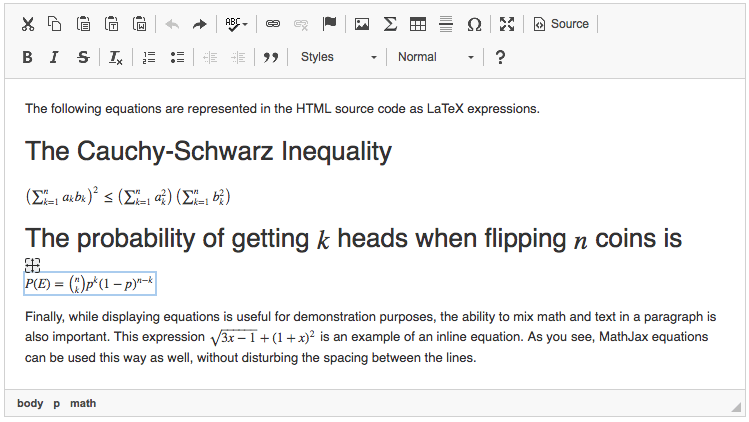#Creating Mathematical Formulas with MathJax

This feature was introduced in CKEditor 4.3. It is provided through an optional plugin that is not included in the CKEditor 4 presets available from the Download site and needs to be added to your custom build with online builder. In order to enable the plugin you need to set the path to the MathJax library first.

The optional Mathematical Formulas plugin allows you to create and modify mathematical equations written in TeX directly in CKEditor 4. TeX content will be automatically replaced by a mathematical formulas widget when you put it in a <span class="math-tex"> element.

New equations can also be inserted into the editor content by using the Math toolbar button and entering TeX code in the plugin dialog window. After you click the OK button, a mathematical formulas widget will be inserted into the editor content.Do note that the equations will be output in plain TeX format with MathJax delimiters, $$ and $$, like in the example below:

<span class="math-tex">$$\sqrt{\frac{a}{b{% raw %}}}{% endraw %}$$</span>


Equations are inserted as widgets, so they have all advantages of widgets, i.e. you can treat the entire equation as one entity and select, delete, or move it with drag and drop in the editor content area as one unit.

## # Setting the Path to MathJax Library

In order to enable the Mathematical Formulas plugin, you need to configure the CKEDITOR.config.mathJaxLib option and set the path to the MathJax library. This value is empty by default, but you can use the free cdnjs service:

config.mathJaxLib = '//cdnjs.cloudflare.com/ajax/libs/mathjax/2.7.4/MathJax.js?config=TeX-AMS_HTML';


You can also use a different path, either a local resource or a different web resource. This configuration option accepts a full or an absolute path. For example:

config.mathJaxLib = 'http:\/\/example.com\/libs\/MathJax.js';


## # Displaying on Target Page

In order to display mathematical formulas on a target page, i.e. the page where content produced by CKEditor 4 will be visible, the target page needs to include the MathJax script. It is advisable to use the same MathJax library version as set in the CKEDITOR.config.mathJaxLib configuration option. For example for the default setting this would be:

<script type="text/javascript" src="https://cdnjs.cloudflare.com/ajax/libs/mathjax/2.7.4/MathJax.js?config=TeX-AMS_HTML"></script>


## # Changing Default Class

You can also modify the default class for <span> elements that are automatically converted into mathematical formulas widgets. Use the CKEDITOR.config.mathJaxClass option to provide a custom class. For example this setting:

config.mathJaxClass = 'equation';


will turn all <span class="equation"> elements into mathematical formulas widgets, including this one:

<span class="equation">$$\sqrt{\frac{a}{b{% raw %}}}{% endraw %}$$</span>


## # Mathematical Formulas Demo

See the working “Creating Mathematical Formulas with MathJax” sample that showcases the Mathematical Formulas plugin with its MathJax widget that supports writing equations in TeX.